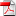# Institut für Thermodynamik der Luft- und Raumfahrt - Universität StuttgartMechanical Engineering - The University of Texas at Austin# boundary layer

The benchmark dataset for the laminar boundary layer is the incompressible Blasius problem. This classic problem goes by several other names, including the u= constant problem, the flat plate problem, the zero-pressure-gradient problem, or the Falkner-Skan m=0 problem. This dataset belongs to the class of problems called the external flow, implying the solution requires the free stream velocity distribution as a boundary condition.

Dataset Name - The Blasius problem is the benchmark dataset s10.dat.txt.

Problem Description - The benchmark laminar boundary layer flow is similar in form to CHMT problem 9.7 (for momentum transfer) and 10.16 (for heat transfer). The Blasius problem requires laminar flow over a flat plate (no surface curvature) with constant surface temperature and constant free stream velocity. The problem description includes:

• u = 15 m/s (the free stream velocity)
• T = 300K (the free stream temperature)
• Ts = 295K (the surface temperature, constant)
• fluid:
• air at 1 atm (101325 Pa)
• properties constant
note: this requires no body force or viscous dissipation effects
• geometry:
• planar
• dimensions: 0.2 m long in the flow direction by 1 m wide
• note: overall length compares to an x-Reynolds number ~ 2 x 105 for Pr=0.7 and Pr=1.0
• start condition: laminar flow at an x-Reynolds number ~ 1000
• end condition: fluid remains laminar (matches a stop x-Reynolds number ~ 2 x 105)

website updated Sept 2010   © 1996-2010 Michael E. Crawford - all rights reserved - website validated for CSS 2.1 and XHTML 1.0 strict at www.w3.org